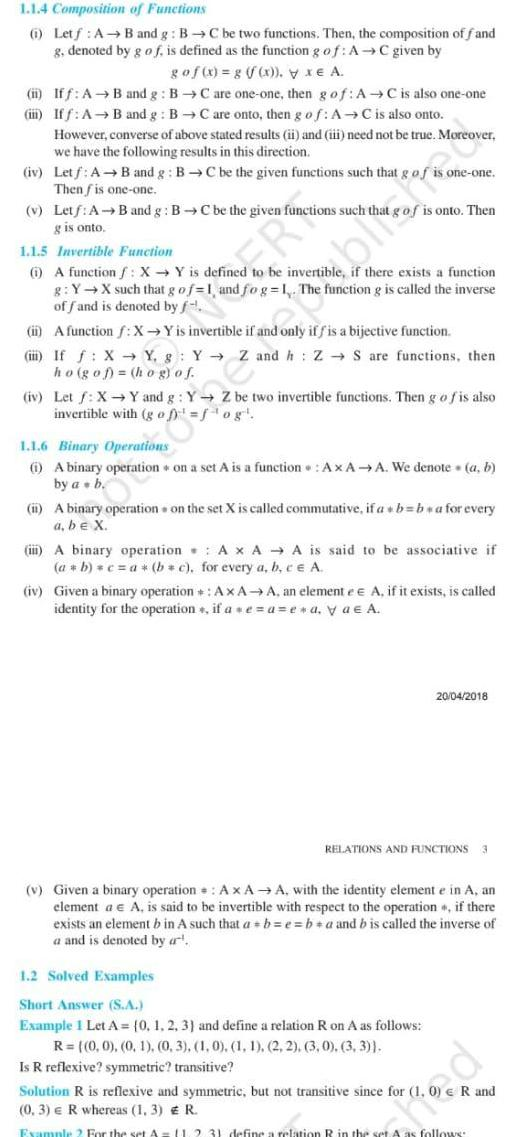Question:

# 1 1 4 Composition of Functions 1 Letf A B and g B C be two

Last updated: 9/19/20231 1 4 Composition of Functions 1 Letf A B and g B C be two functions Then the composition of f and g denoted by g of is defined as the function g of AC given by go f x g y xe A ii Iff A B and g BC are one one then g of A C is also one one iii Iff A B and g BC are onto then g of AC is also onto However converse of above stated results ii and iii need not be true Moreover we have the following results in this direction iv Letf A B and g BC be the given functions such that g of Then fis one one v Letf A B and g BC be the given functions such that g is onto ER 1 1 5 Invertible Function 1 A function f X Y is defined to be invertible if there exists a function g Y X such that g of 1 and fog 1 The function g is called the inverse of fand is denoted by f ii A function f X Y is invertible if and only if f is a bijective function blated one one is onto Then i If f X Y g Y Z and h Z S are functions then ho gof hog of iv Let f X Y and g Y Z be two invertible functions Then g of is also invertible with gof fog 1 1 6 Binary Operations i A binary operation on a set A is a function AxA A We denote a b by a b ii A binary operation on the set X is called commutative if a b ba for every a be X iii A binary operation A x AA is said to be associative if a b c a b c for every a b c e A iv Given a binary operation AxAA an elemente e A if it exists is called identity for the operation if ae a e a ya A 1 2 Solved Examples Short Answer S A Example 1 Let A 0 1 2 3 and define a relation R on A as follows R 0 0 0 1 0 3 1 0 1 1 2 2 3 0 3 3 Is R reflexive symmetric transitive RELATIONS AND FUNCTIONS 3 v Given a binary operation A x AA with the identity element e in A an element a A is said to be invertible with respect to the operation if there exists an element b in A such that a b e ba and b is called the inverse of a and is denoted by a Solution R is reflexive and symmetric but not transitive since for 1 0 3 e R whereas 1 3 Example 2 For the set A 20 04 2018 R and R 11 2 3 define a relation R in the set A as follows